Next: Shot interpolation of the Up: Interpolation Previous: Interpolation

## Theory

First, the shot gathers are sorted into CMP gathers. Therefore, shot interpolation becomes a trace interpolation problem in the CMP domain. The traces to be interpolated are replaced with zeroed traces. Let's calla given CMP gather to be interpolated. The goal is to find a model spacethat minimizes the difference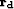between the known data and the modeled data with an HRT operator, i.e.,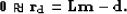(65)
Because missing traces are present in the data, a masking operator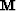is introduced such that the model will only fit the known data as follows:(66)
The masking operator equals one where the data are known, zero otherwise. Solving forin a least-squares sense leads to the following objective function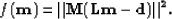(67)

In most cases, the data to be interpolated are aliased, creating strong artifacts in. An efficient way to mitigate these artifacts is by introducing a regularization operator in equation () that will enforce sparseness in the model space. This regularization isolates the strongest events in the model space while ignoring the weakest. To achieve this, a Cauchy regularization Sacchi and Ulrych (1995); Trad et al. (2003) is introduced in equation () as follows:(68)
where n is the size of the model space,is a trade-off parameter between data fitting and model sparseness and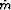is a constant that controls how much damping is applied to the elements of the model space. In practice, bothandare estimated by trial and error.

By definition, introducing the Cauchy norm in equation () makes the problem of findingnon-linear. To take the nonlinearity of the objective function into account,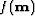is minimized with the quasi-Newton method introduced in Chapter. In practice, this choice leads to satisfying results after a few number of iterations (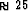).

Once a model is estimated, the interpolated CMP gathersare obtained by forward modeling the data from the estimated model space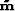and replacing the modeled traces by the known traces as follows:(69)
whereis the identity matrix. This approach preserves the known data exactly. Once the CMP gathers are interpolated, the next step consists in resorting the data into shot gathers.

In the next section, the interpolation technique is applied to the data. This example illustrates that the shots can be effectively interpolated with the radon-based technique.Next: Shot interpolation of the Up: Interpolation Previous: Interpolation
Stanford Exploration Project
5/5/2005